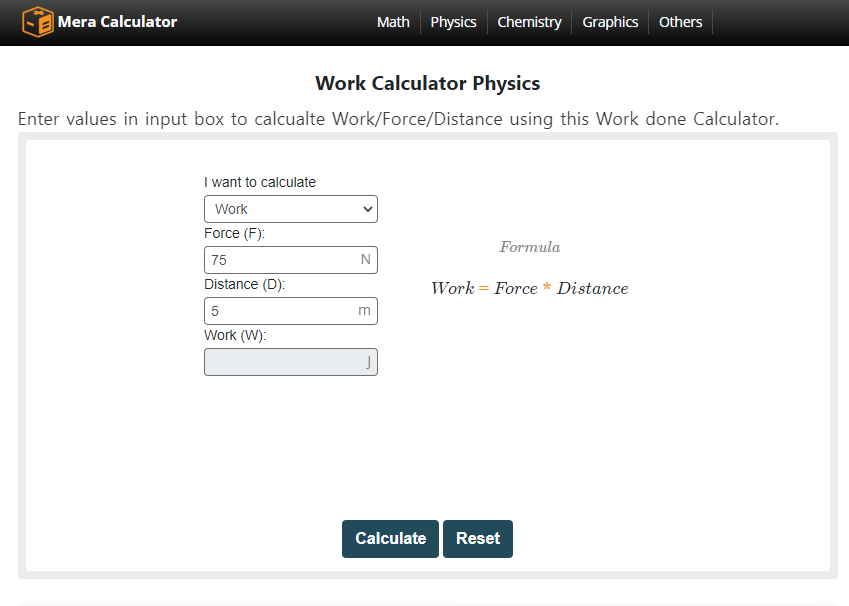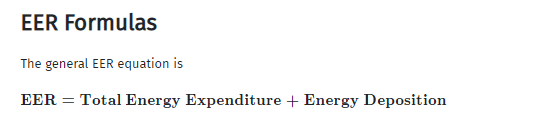When the force moves an object to the distance t is called work done. It is the most common term used in physics or sometimes mathematics.

We all know that work is defined as something that needs mental and physical effort and without any of them, it is not termed as work.

The simplest concept is when we push something or even pull something, we are doing some work so, this is the basic concept.

But we can say that many students are unable to understand this term or they find it a bit tricky or frustrated but this is not true.

Calculating the work done is very simple and easy as well, all you need is to use a formula that is pre-defined by the mathematicians.

The formula is very simple and you don’t need to change anything in the formula, you just need to put the values in it.

We can also use the work done calculator and it will surely make our task easier to solve and understand.The calculator is more reliable as there are fewer chances of calculation mistakes. The calculators for calculating work done by a force are available for free with instant access.

Formula

The formula for calculating the work done is very simple and you just need the value of force and the distance covered.

When you have these two values, you can easily get the work done value from these so, first, you must have these values.

The formula used for calculating is:

Work done= force x distance

This formula is same for the both subjects’ mathematics and physics and you can easily calculate

work done by putting the values in this formula.

There are some derivations or some other concepts that can be helpful in better understanding the concept of work done.

Concept of steam engines

As we all know that in the beginning, we took work by the domestic animals like donkeys and other animals but later we have many more sources.

Steam engines are the very first achievement regarding work done like these engines were used

in various tasks like pumping water from the mines.

The reason for presenting this theory is to make you known about there are many kinds of work and the steam engine was the very first mechanical achievement.

Understanding the term Energy

Energy refers to something that makes us unable to do some work and we can say that without having energy, we cannot even move the lightest object.

The simplest statement to understand the importance of energy is the capacity to do work is known as energy and it is the basic concept.

So, energy is the most basic thing in doing some work, many equations help us calculate the amount of energy required or the amount of energy used.We can easily calculate the energy by using these equations and all you need is to put the values in the equation and you are all set to calculate.

Another method of calculating estimate Energy Required is to use an online EER calculator.

We don’t have only one kind of energy but we have various types of energies that are termed chemical energy, mechanical energy, heat energy, and many more.

Bottom line

Using equations is not that much difficult unless we find a complex equation but we can say that the equation of work done is the simplest.

The thing you need to do is putting the values in the equation and you can easily get the amount of work done.

As we mentioned above the concept of work done and is enough for understanding the whole scenario.

We also discussed one of the most important terms energy that is needed to mention when it comes to the understanding of the work done.

We also discussed the types of energies and you can easily convert one energy into the other so, it is not that much difficult.